# Custom Tables

This article will explain what custom data tables are, why they are useful, how to set them up, and how to use them from within P3L.

### What are custom tables?

Custom tables are data that you can create and store in Paperless Parts. Custom tables are similar to CSV files, but with slightly more rigid enforcement of column names and row values.

### Why are custom tables useful?

Custom tables are useful because it allows you to create small or large collections of data that your pricing formulas can reference. From within P3L, you can perform filtering actions on any columns in your data table to look up pertinent information based on the characteristics of the current part you are quoting. As long as you can collect your data into a clean CSV, you can use it to influence pricing. A common use case for custom tables is to perform look-ups for material pricing/properties given the material and geometric properties of a part.

### How do I set up my custom tables?

You can manage your custom data tables from the processes page. Additionally, you can create, update, and delete custom tables using our open API. Every table must have a unique alpha-numeric name. Every table will have a discrete number of type-enforced columns. Tables can have as many rows as you like. Columns must have alpha-numeric names WITHOUT spaces and must be one of three types: boolean, numeric, or string. When adding or updating rows, these types will be enforced. When performing look ups on these tables, the conditional values will also by type-enforced.

Look for the "Tables" tab in the processes page. Here you can create your new tables: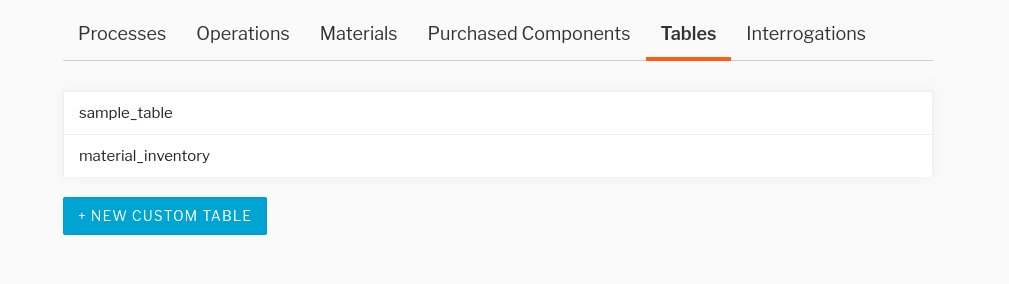Once you create a new table on this page, you can click on any row to configure the details of a table.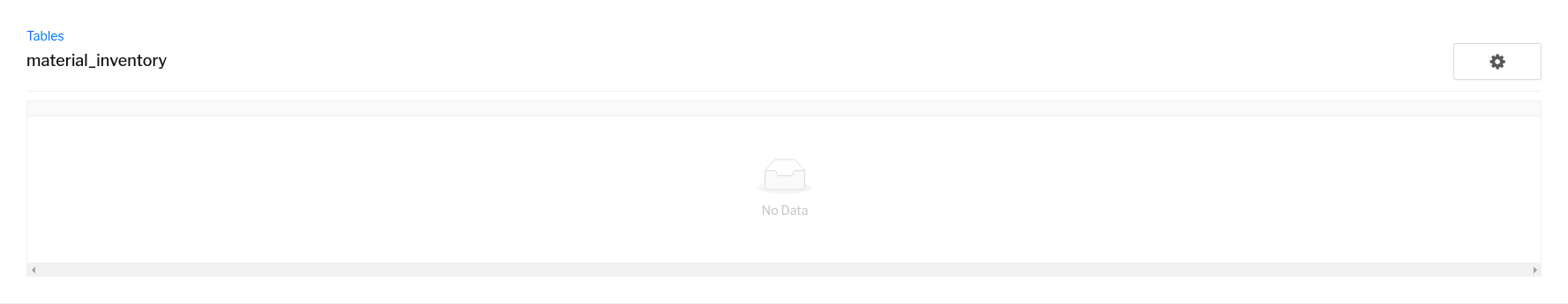To add data to your custom table, you need to provide a configuration csv and a data csv file. The configuration file will have three columns: column_name and value_type and is_for_unique_key. The column_name is the alpha-numeric name of the column that will be used to perform lookups from within your P3L formulas. The value_type column can be one of three values: boolean, numeric, or string. The is_for_unique_key column is a boolean (TRUE or FALSE). The data file you upload must have matching column names to the ones specified in the config file. Additionally, the values for every cell in your data csv must be castable to the value_type specified for that column in the config csv. Your csv files will not upload properly if any issues are found with format, column names, or typing. To perform this action, click the "Update Table" option from the gear drop down in the top right.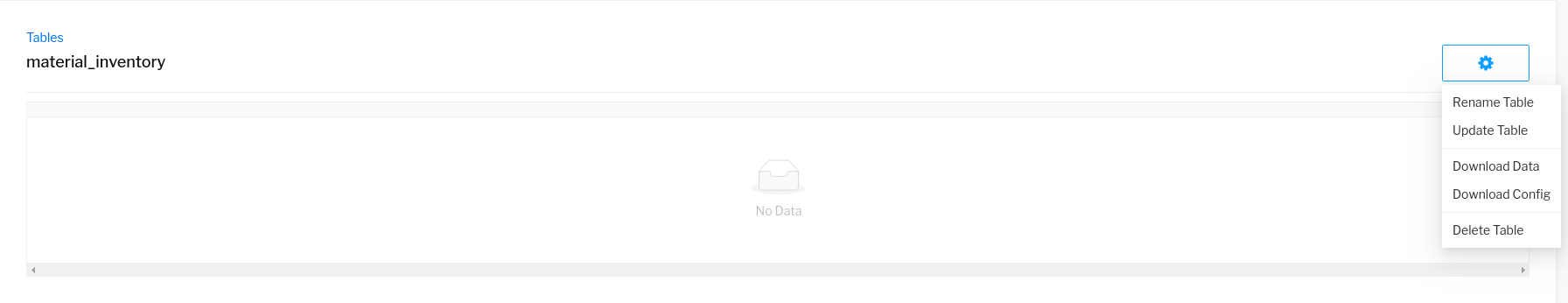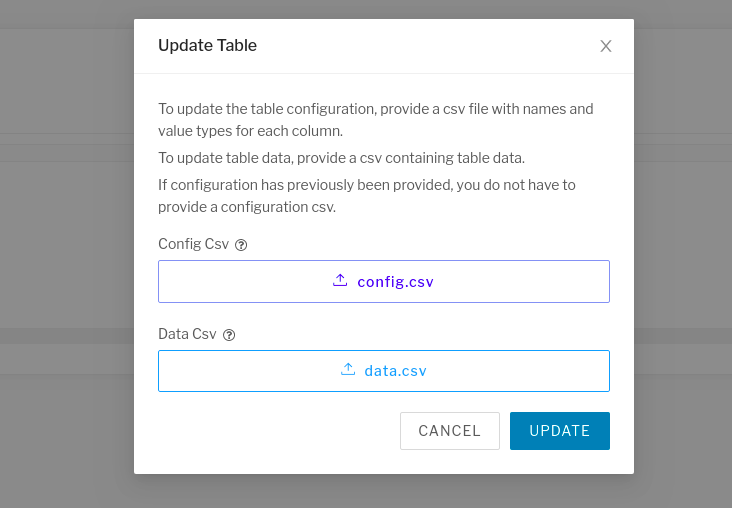After submitting, you should now be able to view your table data.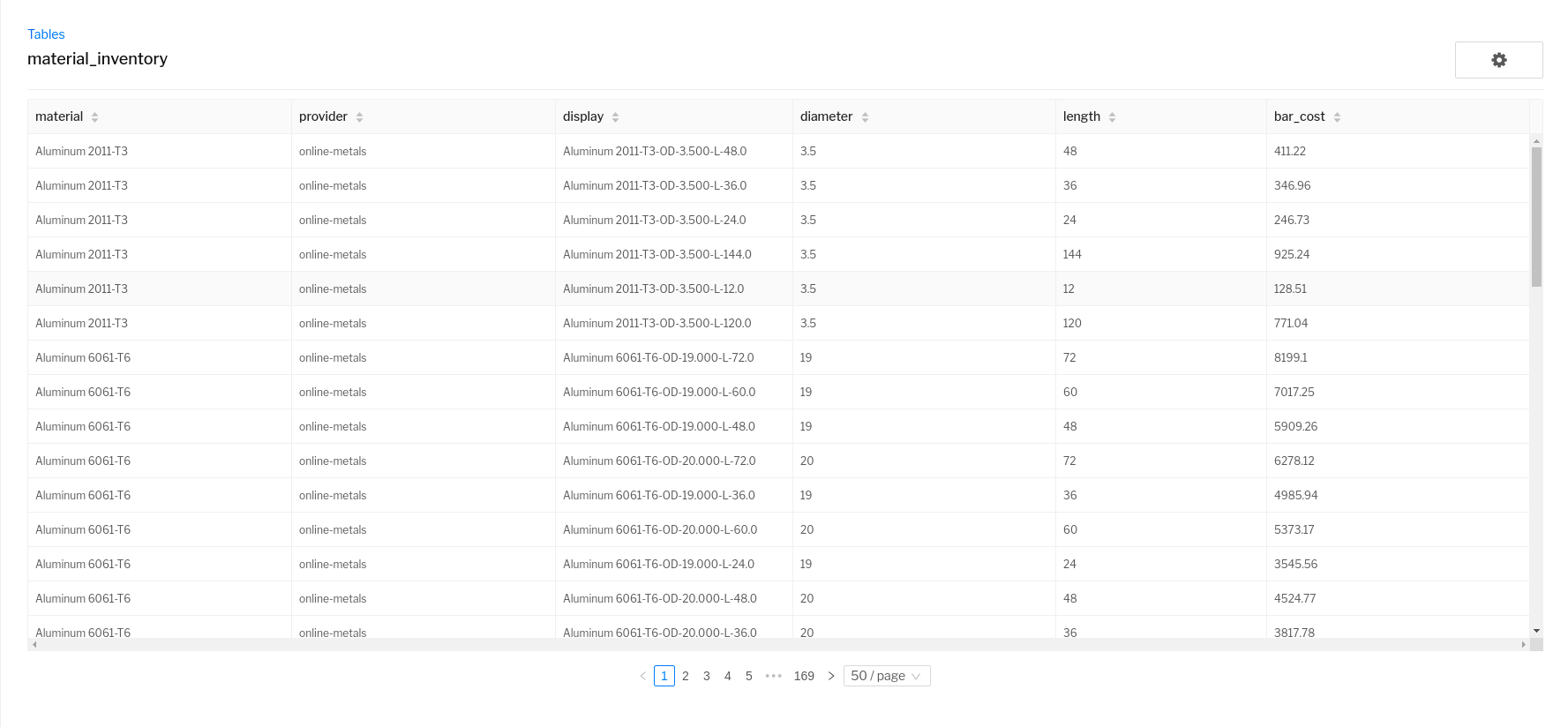To edit the configuration or data in your table, navigate to the gear to upload new csv files. (Note that if you have previously provided a configuration file and you just want to update your data, you can provide just a csv with the new row data. You do not have to provide a configuration file again. But note that only columns with matching column names to the config file will actually populate to your custom table.)

Additionally, in the gear drop down, you can download csv files for both the table configuration and table data. Furthermore, once you have set up P3L formulas that reference this table, you will be able to quickly view those from the action gear drop down.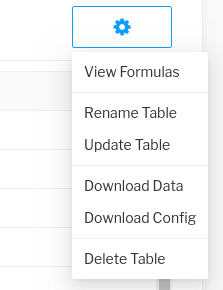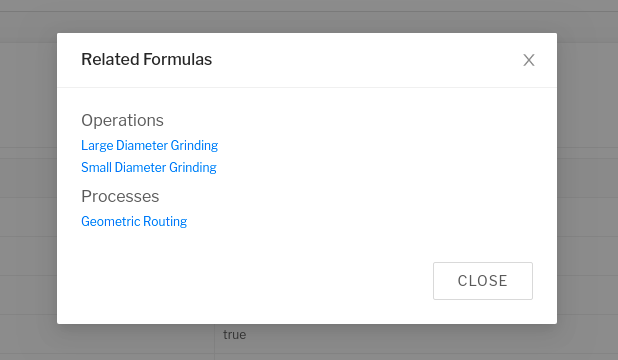### How do I use my custom tables in my pricing formulas?

Accessing information from your custom tables is available in both operation-level and process-level P3L. Similar to variables using the `var` function from operation P3L, you create a `table_var` that references a specific table along with any filtering and ordering conditions:

`table_var(name: str, description: str, table_name: str, filters: List[filter | exclude], order_by: List[str], display_column_name: str, frozen: bool = True, quantity_specific: bool = False) -> Union[Optional[TableRow], TableVariable]`

This declares a table variable with the given name and description from the specified table with the name table_name. The filters input should always be the output of a `create_filter()` function call (see below). The order_by input should always be the output of a `create_order_by()` function call (see below). The filters and order_by will dictate how the table is queried and the result is ordered. The display_column_name is the name of the column that will be used as the display value for the row in the quoting interface. The frozen input is a Boolean field that dictates whether this table variable can be updated dynamically or is static, and it will affect the type of object that is returned from the function. The frozen input defaults to `True`. The quantity_specific input is a Boolean that dictates whether the query to the custom table is performed for each quantity or once for all quantities and whether the variable value is independent, displayed, override-able for each quantity break. This input defaults to `False`. This is an advanced concept. Check out our article on quantity-specific variables for more information.

If `frozen=True` (or optionally not specified as it defaults to `True`), the output of this function will be the FIRST row that satisfies the query filters adhering to the ordering specified by order_by. The outputted row will be an instance of a `TableRow`. You can use dot notation to access the columns of a `TableRow`. A `TableRow` has additional methods explained below. If there are no rows found that satisfy the filters, the output will be `None`. If `frozen=False`, a `TableVariable` object will be returned. This is an object that contains a reference to the rows that satisfy the filter conditions and a value property you can dynamically update from the list of rows. You can dynamically update the `value` for the `TableVariable` using several methods explained below (e.g. `select_first()`). You update the `value` by looking for the `TableRow` instance from the `rows` property. Both the `value` and `rows` property are accessible via dot notation. The `value` is either `None` or a `TableRow` depending on if there are any rows to satisfy the filters and if your selection criteria exists. Specifying `frozen=False` is particularly useful if you want to dynamically select values based on quantity-driven variables. `TableVariable` objects become frozen after you apply a selection using a selector method. Overrides applied in the UI take affect at the location you freeze the `TableVariable`. See below for more details on `TableVariable`

Similar to standard variables from the `var` function, table variables can be overridden in the quoting interface. See below for examples of usage.

NOTE: Only up to the first 200 rows satisfying filter conditions will be returned from this function call.

`table_lookup(table_name: str, filters: List[filter | exclude], order_by: List[str], quantity_specific: bool = False) -> P3LList[TableRow]`

This creates a lookup to the specified table_name. This works in a similar fashion to `table_var`, except it returns a P3LList of all rows that satisfy the filters in the order specified by order_by. The quantity_specific is a Boolean that defaults to `False` that dictates whether the query to the custom table will be performed for each quantity, or once across all quantities. This is useful when your filter conditions depend on the `part.qty` value in some way. Checkout our article on quantity-specific variables for more details.

This WILL NOT create a new variable instance that is override-able at quote time in the live pricing interface. This is used as a utility to collect larger chunks of data from your custom tables to work with in pricing programs. Using a `table_lookup` instead of a `table_var` is advisable in two situations. The first is when you need to extract information from a table without wanting to line it out as a variable in the UI. The second is when you want to manipulate larger quantities of data. A `table_var` will only allow you to interact with up to 200 rows of data. A `table_lookup` allows you to work with up to 10,000 rows of data. The reason we can provide more rows to work with is because we do not need to store these rows for interaction in the live pricing UI. A few examples where `table_lookup` is a better data structure than `table_var` are when trying to apply tooling to a set features, applying removal rates based on material, establishing routing based on historical pricing tables extracted from your ERP system, and when collecting options to dynamically add to a drop down variable. Searching for results within the drop-down variable can return a max of 50 results. See below for examples of usage.

NOTE: Only up to the first 10,000 rows satisfying the filter conditions will be returned from this function call.

`create_filter(*args: [filter | exclude])`

This function takes an arbitrary number of inputs of the form of a `filter()` or an `exclude()` function call. See below for examples of usage.

`filter(column_name: str, condition: str, value)`

This creates an inclusive filter based on the column_name, the condition, and the value. This will include all rows that satisfy the condition described by the inputs. See below for information on supported condition types. The value needs to match the characteristic type of the column in the table specified by the column_name. The value can be dynamic such as the properties in the `part` object or upstream dynamic variables.

`exclude(column_name: str, condition: str, value)`

This creates an  exclusive filter based on the column_name, the condition, and the value. This will exclude all rows that satisfy the condition described by the inputs. See below for information on supported condition types. The value needs to match the characteristic type of the column in the table specified by the column_name. The value can be dynamic such as the properties in the `part` object or upstream dynamic variables.

`create_order_by(*args: str)`

This function takes an arbitrary number of string arguments that are column names on the custom table. This will inform how to sort the rows that satisfy the filters provided to the table variable. Establishing how to order the rows is important because the output of the `table_var` function is the first row that satisfies the filter after applying ordering. When specifying a column name to sort by, it will be in ascending order. However, if you want to order in descending fashion, simply add a "-" before the input. For example, to order rows by a column named `diameter` so that the row with the smallest diameter value is returned first, you would do `create_order_by('diameter')`. To order rows by a column named `diameter` so that the row with the greatest diameter is returned first, you would do `create_order_by('-diameter')`. This also supports multiple column ordering, so something like `create_order_by('diameter', 'length', '-thickness')` is valid.

`create_range(a, b)`

This function takes numeric-like arguments  a and b and creates a range to use as the value for a `filter()` or `exclude()` function call with `'range'` as the specified condition. These inputs can be variables or literals. See the table below for

#### Filter Conditions

The conditions below can be used with both the *filter()* and *exclude()* functions. Lets assume we have a custom table with column names  material (string), diameter (numeric), and length (numeric).

 Condition Compatible Types Usage = Boolean, numeric, string `filter('material', '=', 'Aluminum 6061-T6')` > numeric, string `exclude('length', '>', 10)` >= numeric, string `filter('diameter', '>=', 5)` < numeric, string `exclude('length', '<', 10)` <= numeric, string `filter('diameter', '<=', 5)` range numeric `filter('diameter', 'range', create_range(5, 6))` contains string `filter('material', 'contains', 'Aluminum')`

#### Static `table_var` Usage

As mentioned above, the output of a `table_var` call can change based on whether you specify `frozen=True` or `frozen=False` (default `True`). If `frozen=False` (or not specified as it defaults to `False`), the output is a `TableRow` (or `None` if no rows match the filter. This is what we call a static table variable. Based on the schema you establish for your custom table in the config file, you can access the different values of a `TableRow` using "dot" notation. Additionally, the `row_number` is also available on every returned `TableRow` instance as well as the properties from the table. For example, to access the properties from our sample material_inventory table we created, we would use `row.material`, `row.diameter`, `row.row_number`, etc. Because you must use dot notation in, your column names must be alphanumeric and cannot have spaces or start with numbers. Additionally, a `TableRow` instance has three other utility methods to cast its keys, values, and items into `P3LList` format (check out P3L Lists article for more information on this data structure). This can be useful when using custom tables with drop down variables when you want to store dynamic drop down options in a custom table. Below, the code block shows how to use the dot notation and the list method helpers. Let's assume the returned row is the top row from the table screenshot above.

```row = table_var(
'Material Selection',
'look up for dynamic cylindrical stock selection',
'material_inventory',
create_filter(
filter('material', '=', 'Aluminum 2011-T3'),
filter('bar_cost', '=', 411.22),
),
create_order_by('material'),
'display'
)

row.row_number  # integer of row number (built in)
row.material  # string of material
row.diameter  # float of diameter

# cast the keys of the row to a p3l list
l1 = row.to_keys_list()
l1.sort(lambda x: x)
#  l1 == ['bar_cost', 'diameter', 'display', 'length', 'material', 'provider', 'row_number']
l2 = row.to_values_list()
#  l2 == [411.22, 3.5, 'Aluminum 2011-T3-OD-3.500-L-48.0', 48, 'Aluminum 2011-T3', 'online-metals', 0]
l3 = row.to_list()
"""
l3 = [
{'key': 'bar_cost', 'value': 411.12},
{'key': 'diameter', 'value': 3.5},
{'key': 'display', 'value': 'Aluminum 2011-T3-OD-3.500-L-48.0'},
{'key': 'length', 'value': 48},
{'key': 'material', 'value': 'Aluminum 2011-T3'},
{'key': 'provider', 'value': 'online-metals'},
{'key': 'row_number', 'value': 0},
]
"""
```

#### Dynamic `table_var` Usage

When you specify `frozen=False` into the `table_var` function, a `TableVariable` object is returned. This is what we call a dynamic table variable. This object allows you to interact with all of the rows that satisfy the filter condition instead of just the first row when `frozen=True`. Also, this object allows you to select the value the table variable will have after you have performed the query. You select the value by searching for the row you want within the rows that satisfy the filter requirements. Once you select a value, the variable then becomes frozen, and if an override is applied in the live pricing UI, this is where the override takes effect.

#### `TableVariable` Properties

A `TableVariable` has two properties accessible via "dot" notation. Let's assume we initialized a table variable using the `table_var` function as `tv`:

 Property Description `tv.value` Returns the currently selected value for the table variable. Either `None` or an instance of a `TableRow` object. This can be updated using select methods. `tv.rows` Returns a `P3LList` copy of `TableRow` objects that satisfy the filter conditions in the order specified by the `order_by` conditions.

#### `TableVariable` Methods

All of these methods pertain to selecting a value for the `TableVariable`. After performing any of these methods, the `tv.value` property will be updated to the object you have selected. If any overrides are applied in the live pricing UI, they will take affect at the moment of selection. Additionally, after calling any of these methods, the object will become frozen. You cannot perform redundant select actions on a frozen `TableVariable`. The program will throw an error if a redundant select action is performed.

`select_first()`

Sets the first row from `tv.rows` as the value based on ordering specified by the `order_by` conditions. If there are not any rows because no rows satisfy the filter conditions, this method will safely set the value to `None`. This method is useful for when you cannot find a row to satisfy your custom logic and simply want to freeze the variable in place.

`select_last()`

Sets the last row from `tv.rows` as the value based on ordering specified by the `order_by` conditions. If there are not any rows because no rows satisfy the filter conditions, this method will safely set the value to `None`

`select_by_row(row: TableRow)`

Sets the input `row` as the value. If the row you specify is not found in `tv.rows`, this method will safely set the value to `None`.

`select_by_row_number(row_number: int)`

Searches for the `TableRow` object with the corresponding `row_number` in the `tv.rows` array. If a row is found with the inputted `row_number` it will set the value to that row, otherwise, it will set the value to `None`.

Here is a code block explaining basic usage for dynamic table variables. Let's query for all of the Aluminum 2011-T3 rows we see in the sample table screenshot above.

```tv = table_var(
'Material Selection',
'look up for dynamic cylindrical stock selection',
'material_inventory',
create_filter(
filter('material', '=', 'Aluminum 2011-T3'),
),
create_order_by('length'),
'display',
frozen=False,
)

tv.rows  # p3l list copy of rows
first_row = tv.rows
last_row = tv.rows[-1]
last_row.material  # "Aluminum 2011-T3"
last_row.diameter  # 3.5
last_row.length  # 144

# select first entity -> freezes variable, overrides are applied at moment of selection
tv.select_first()
tv.value.material  # "Aluminum 2011-T3"
tv.value.diameter  # 3.5
tv.value.length  # 12

# ...assume we have a new variable "tv" that we can select
# NOTE: you can only perform one selection on a table_var, selecting a frozen variable
# will throw an error
# select last entity -> freezes variable
tv.select_last()
tv.value.material  # "Aluminum 2011-T3"
tv.value.diameter  # 3.5
tv.value.length  # 144

# ...assume we have a new variable "tv" that we can select
# select first entity by row
tv.select_by_row(tv.rows)
tv.value.material  # "Aluminum 2011-T3"
tv.value.diameter  # 3.5
tv.value.length  # 24

# ...assume we have a new variable "tv" that we can select
# select first entity by row number
tv.select_by_row_number(tv.rows.row_number)
tv.value.material  # "Aluminum 2011-T3"
tv.value.diameter  # 3.5
tv.value.length  # 24
```

#### `table_lookup` Basic Usage

As explained above, `table_lookup` operates similarlar to a `table_var`. Using this function will return all of the rows that satisfy the filter conditions ordered by the `order_by` conditions. Using this function WILL NOT create a variable instance that is override-able in the live pricing UI at quote time. Use `table_lookup` over `table_var` when you do not want to line out a variable and/or when you want to work with larger amounts of data. When using `table_var` you can only collect 200 rows that satisfy the filter conditions. When using `table_lookup` you can collect up to 10,000 rows of data. `table_lookup` returns a P3LList of `TableRow` objects. Let's perform a `table_lookup` to the material_inventory table to grab all of the rows that have the name "Aluminum 2011-T3":

```rows = table_lookup(
'material_inventory',
create_filter(
filter('material', '=', 'Aluminum 2011-T3'),
),
create_order_by('length'),
)

len(rows)  # 6
# access rows via dot notation
rows.material  # "Aluminum 2011-T3
rows.diameter  # 3.5
rows.length  # 12

# ...iterate thru rows to find information you want
for row in iterate(rows):
if row.length > 36:
do_something = 1

# use lambdas on rows to sort/filter/map
l1 = rows.copy().filter(lambda x: x.length >= 36).map(lambda x: x.length).sort(lambda x: -x.length)
# l1 == [144, 120, 48, 36]
```

#### `table_var` Example - Select Rod Stock for Material Operation

Let's walk through an example that references the material_inventory table above. For a lathe process, let's set up a material op that looks to the custom table to find the cheapest bar in terms of cost per length. We need to make sure this bar is large enough to fit at least one unit, so our rod stock must have a diameter and length greater than or equal to our final part geometry. We will then sort our result to ensure we grab the row with the smallest diameter, greatest length (as the greater the length the cheaper it will be in terms of cost per length), and the smallest cost. Based on our selection, we will then determine a parts per rod, and resolve a cost based on how many units we have to make. We then store values like rod length and diameter in the workpiece so we can reference those in downstream operations.

```units_in()
lathe = analyze_lathe()

part_diameter = var('Part Diameter', 0, '', number, frozen=False)
part_diameter.freeze()

part_length = var('Part Length', 0, '', number, frozen=False)
part_length.update(lathe.stock_length)
part_length.freeze()

row = table_var(
'Material Selection',
'look up for dynamic cylindrical stock selection',
'material_inventory',
create_filter(
filter('material', '=', part.material),
filter('diameter', '>=', part_diameter),
filter('length', '>=', part_length),
),
create_order_by('diameter', '-length', 'bar_cost'),
'display',
)

if row:
set_operation_name(row.display)

rod_cost = var('Rod Cost', 0, '', currency, frozen=False)
if row:
rod_cost.update(row.bar_cost)
rod_cost.freeze()

rod_diameter = var('Rod Diameter', 0, '', number, frozen=False)
if row:
rod_diameter.update(row.diameter)
rod_diameter.freeze()

rod_length = var('Rod Length', 0, '', number, frozen=False)
if row:
rod_length.update(row.length)
rod_length.freeze()

cut_width = var('Cut Width', 0.25, 'width of cut on inches for saw', number)

parts_per_rod = var('Parts Per Rod', 0, '', number, frozen=False)
parts_per_rod.update(floor(rod_length / (part_length + cut_width)))
parts_per_rod.freeze()

rod_count = ceil(part.qty / parts_per_rod)

PRICE = rod_count * rod_cost
DAYS = 0

set_workpiece_value('rod_diameter', rod_diameter)
set_workpiece_value('rod_length', rod_length)
```

Check out the article on quantity-specific variables to see how we can extend/modify this example to select the best rod for individual quantities in the event you are quoting both low and high quantity jobs.

#### `table_var` Basic Example - Laser Cut Rates

Let's walk through another example so we get an even better sense for how to take advantage of custom tables. Let's say we have a laser cutting operation for a sheet metal process. For our laser cutter, we have pierce times and cut rates based on the type of the material and the thickness of the material. So, let's imagine we set up a custom table with the name 'laser_cut_rates'. The columns (types) for this custom table are material_family (string), thickness (numeric), cut_rate (numeric), and pierce_time (numeric). That custom table would look something like the following: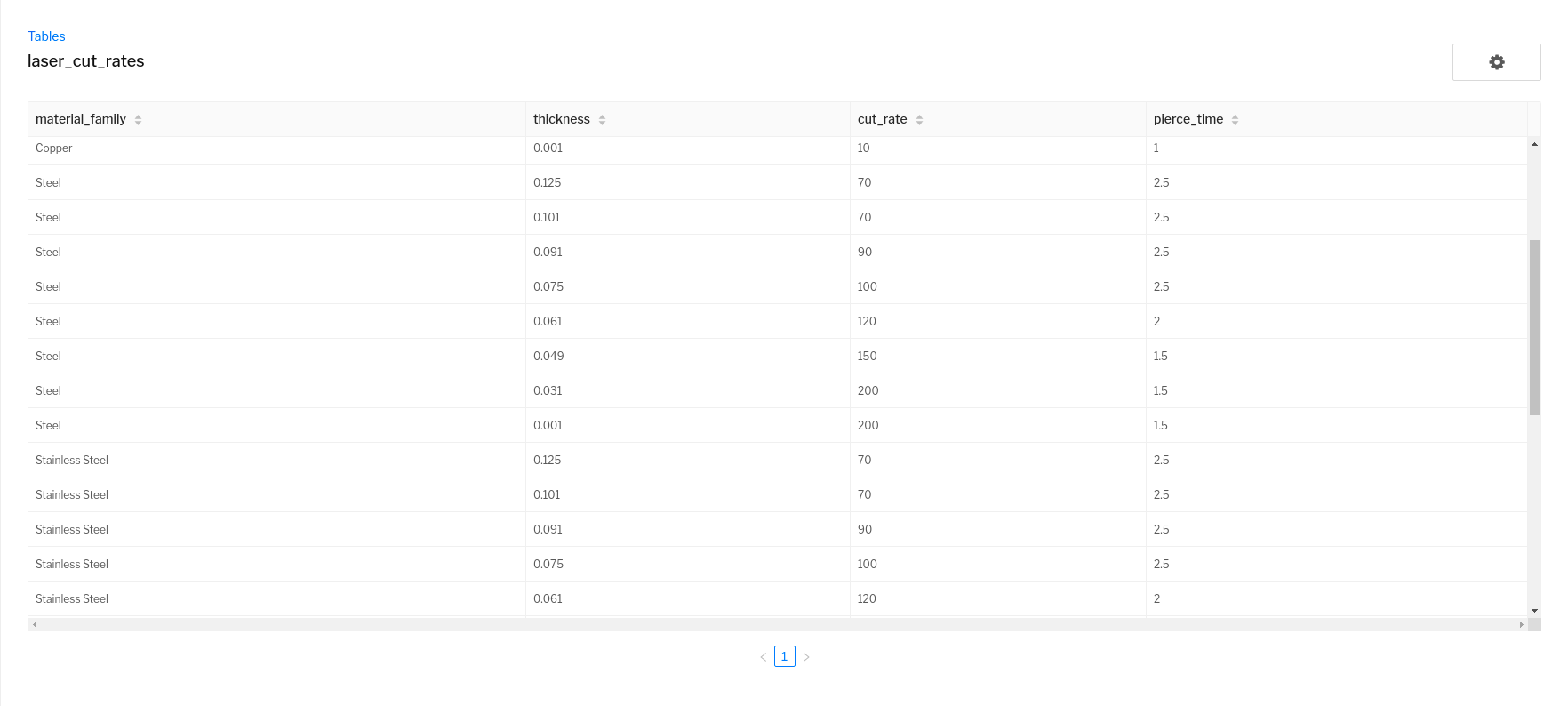The operation below would query that custom table based on the material family and the detected thickness from sheet metal interrogation. We then determine a runtime based on the total number of pierces and cut length extracted by the Paperless sheet metal interrogation.

```units_in()

sm = analyze_sheet_metal()

thickness = var('Thickness', 0, 'Thickness of material', number, frozen=False)
thickness.update(sm.thickness)
thickness.freeze()

# query table for thickness plus or minus one gauge width
row = table_var(
'Material Selection',
'Cutting properties for thickness and material family',
'laser_cut_rates',
create_filter(
filter('material_family', '=', part.material_family),
filter('thickness', '>', thickness),
),
create_order_by('thickness', '-cut_rate'),
'cut_rate'
)

cut_rate = var('Cut Rate', 0, 'inches per minute', number, frozen=False)
if row:
cut_rate.update(row.cut_rate)
cut_rate.freeze()

pierce_time = var('Pierce Time', 0, 'pierce time in seconds', number, frozen=False)
if row:
pierce_time.update(row.pierce_time)
pierce_time.freeze()

total_pierce_seconds = sm.pierce_count * pierce_time
total_travel_time = sm.total_cut_length / cut_rate

runtime = var('runtime', 0, 'runtime in hours', number, frozen=False)
runtime.update(total_pierce_seconds / 3600 + total_travel_time / 60)
runtime.freeze()

rate = var('Rate', 50, '\$/hr', currency)

PRICE = runtime * rate * part.qty
DAYS = 0
```

Custom tables are going to be useful when you need to interact with some type of look up table that has more than a few rows. Additionally, interacting with custom tables through the `table_var` P3L function is very useful for look ups that often need to be overridden at quote time.

#### `table_lookup` Basic Example - Punch Tooling

Let's look at an example where using a `table_lookup` would be more effective than using a `table_var`. Let's explore the situation where you have a punch press, and you have a discrete list of tools to hit different cutout geometries. Let's create an operation that checks for available tooling to accomplish the single hit features on a sheet metal part. The way we will accomplish this is by collecting all the rows from our tooling table and then manually filter these rows in our pricing formula to attempt to find a tool that can be used to hit a feature. We will combine custom tables, feature iteration, and P3LList functionality to make all of this work. Let's say we have set up a table called 'punch_tooling'. The columns (types) for this custom table are shape (string), length (numeric), width (numeric), and radius (numeric). That custom table would look something like the following: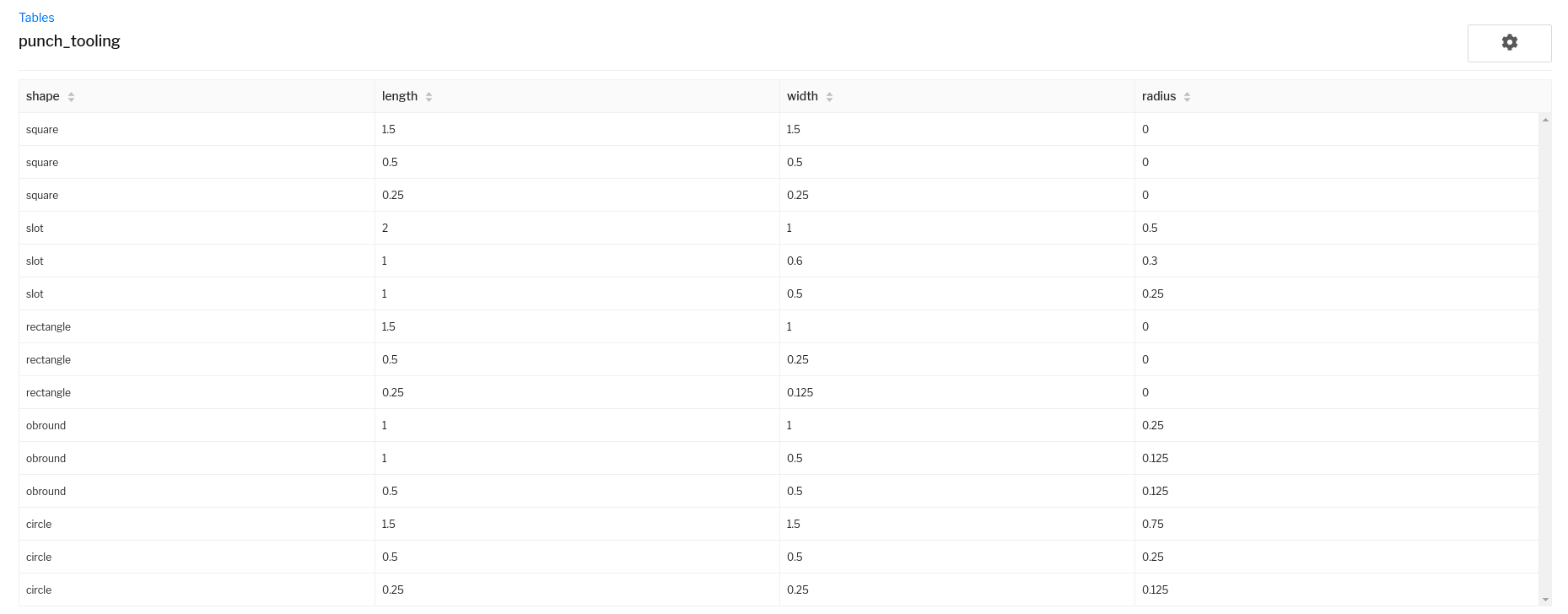Now let's write the operation formula, and collect variables for each type of single hit feature that we cannot hit because we lack the tooling.

```units_in()
sm = analyze_sheet_metal()

rows = table_lookup('punch_tooling', create_filter(), create_order_by())

mct = 0
for f in iterate(sm.features.filter(lambda x: x.name == 'circular_punch')):
applicable_tools = rows.copy().filter(
)
if not applicable_tools:
mct += 1

mot = 0
for f in iterate(sm.features.filter(lambda x: x.name == 'obround_punch')):
props = f.properties
applicable_tools = rows.copy().filter(
lambda x: x.shape == 'obround' and is_close(x.radius, props.radius) and is_close(x.length, props.side_length) and is_close(x.width, props.side_width)
)
if not applicable_tools:
mot += 1

mrt = 0
for f in iterate(sm.features.filter(lambda x: x.name == 'rectangular_punch')):
props = f.properties
applicable_tools = rows.copy().filter(
lambda x: x.shape == 'rectangle' and is_close(x.length, props.side_length) and is_close(x.width, props.side_width)
)
if not applicable_tools:
mrt += 1

mslt = 0
for f in iterate(sm.features.filter(lambda x: x.name == 'slot_punch')):
props = f.properties
applicable_tools = rows.copy().filter(
)
if not applicable_tools:
mslt += 1

msqt = 0
for f in iterate(sm.features.filter(lambda x: x.name == 'square_punch')):
props = f.properties
applicable_tools = rows.copy().filter(
lambda x: x.shape == 'square' and is_close(x.length, props.side_length)
)
if not applicable_tools:
msqt += 1

v1 = var('Circ Missing', 0, '', number, frozen=False)
v1.update(mct)
v1.freeze()
v2 = var('Obround Missing', 0, '', number, frozen=False)
v2.update(mot)
v2.freeze()
v3 = var('Rect Missing', 0, '', number, frozen=False)
v3.update(mrt)
v3.freeze()
v4 = var('Slot Missing', 0, '', number, frozen=False)
v4.update(mslt)
v4.freeze()
v5 = var('Square Missing', 0, '', number, frozen=False)
v5.update(msqt)
v5.freeze()

# name operation for an obvious indication if we have tooling
if v1 > 0 or v2 > 0 or v3 > 0 or v4 > 0 or v5 > 0:
set_operation_name('Missing Tooling for {} features'.format(v1 + v2 + v3 + v4 + v5))
else:
set_operation_name('Tooling Good')
```

The strategy employed in this example can be used similarly for situations like applying different removal rates for milled features depending on their volume, depth, etc.

#### Custom Table Advanced Strategy - Creating Custom Drop Down Forms

##### Options as Row Values

Drop down variables are an awesome way to organize and select information in your pricing formulas. Check out the article on drop down variables for more information on how to work with these variable types. To unlock the power of this variable type even further, you can leverage performing table lookups and creating table variables to populate the options of drop down variables. The simplest way to implement this type of functionality is using a table lookup to a custom table that has options specified a column names. Let's set up a custom table with the name drop_down_inputs that looks like the following: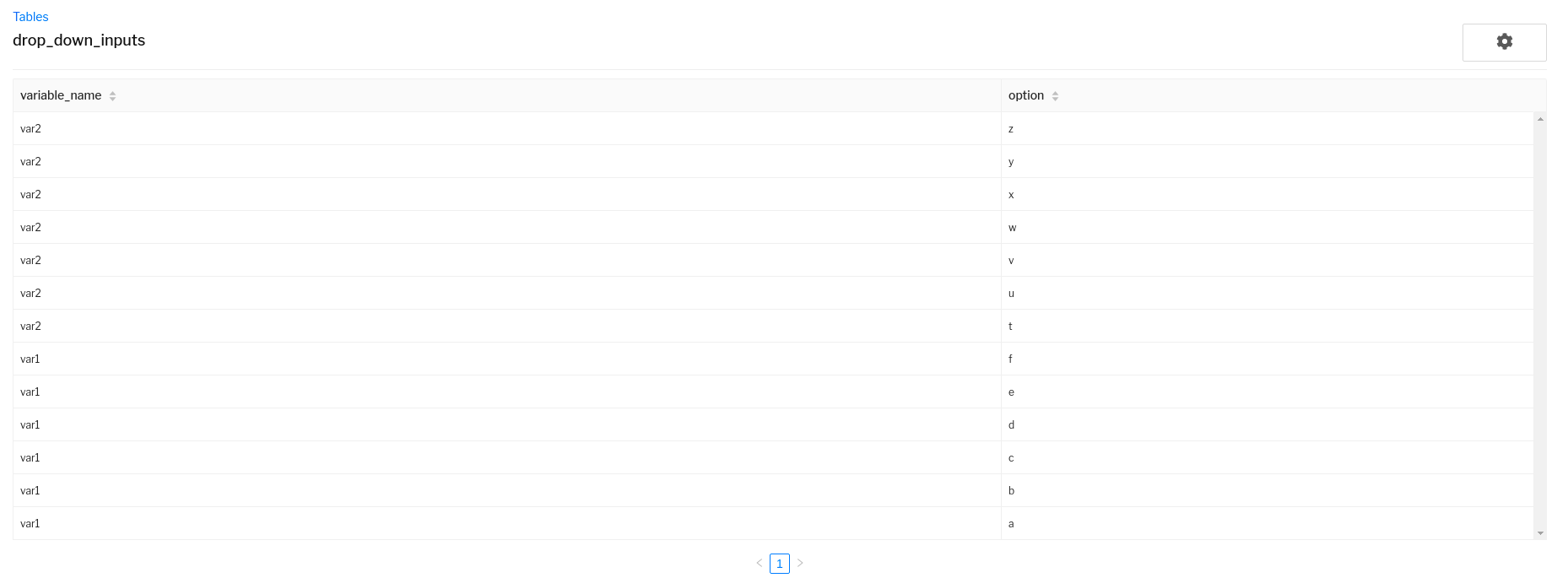Now lets create a simple operation formula with two drop down variables called "var1" and "var2" that references the options from this custom table.

```option_rows = table_lookup('drop_down_inputs', create_filter(), create_order_by())

var1_options = option_rows.copy().filter(lambda x: x.variable_name == 'var1').map(lambda x: x.option).sort(lambda x: x)
var2_options = option_rows.copy().filter(lambda x: x.variable_name == 'var2').map(lambda x: x.option).sort(lambda x: x)

var1 = drop_down_var('var1', 'NONE', create_list(), '', string, frozen=False)
if var1_options:
var1.clear_options()
var1.update_options(var1_options)
var1.select_option(var1_options)
else:
var1.select_default()

var2 = drop_down_var('var2', 'NONE', create_list(), '', string, frozen=False)
if var2_options:
var2.clear_options()
var2.update_options(var2_options)
var2.select_option(var2_options)
else:
var2.select_default()
```

Here is what the resulting formula looks like in the live pricing UI: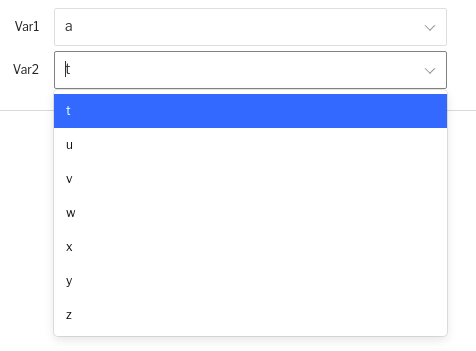##### Options as Comma Separated Strings w/ Default

Another way to implement custom form logic is to specify the options inputs as comma separated strings. This requires a bit more overhead in our P3L, but presents itself more concisely in the table display and allows us to specify things like a default value. Let's set up a custom table called drop_down_options_as_css that looks like this: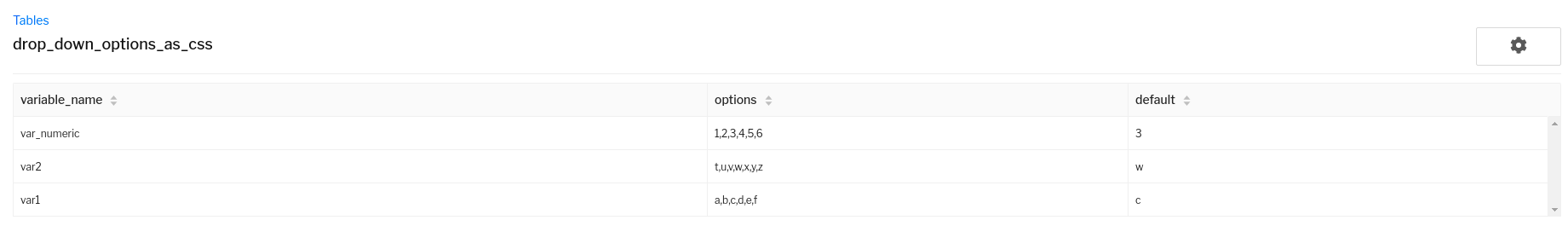Note that each column type will be string even though the `var_numeric` options and defaults are numeric. When we add options to drop down variables, they will be casted to the type specified in the variable declaration. So as long as the strings are castable to floats, they are totally valid inputs to the `drop_down_var` when the value_type input is either `number` or `currency`

To then interact with this table to construct our drop down options, we will implement the following operation code:

```option_rows = table_lookup('drop_down_options_as_css', create_filter(), create_order_by())

var1_rows = option_rows.copy().filter(lambda x: x.variable_name == 'var1')
var2_rows = option_rows.copy().filter(lambda x: x.variable_name == 'var2')
var_numeric_rows = option_rows.copy().filter(lambda x: x.variable_name == 'var_numeric')

var1 = drop_down_var('var1', 'NONE', create_list(), '', string, frozen=False)
if var1_rows:
var1.clear_options()
row = var1_rows
options = row.options.split(',')
var1.update_options(options)
var1.select_option(row.default)
else:
var1.select_default()

var2 = drop_down_var('var2', 'NONE', create_list(), '', string, frozen=False)
if var2_rows:
var2.clear_options()
row = var2_rows
options = row.options.split(',')
var2.update_options(options)
var2.select_option(row.default)
else:
var2.select_default()

var_numeric = drop_down_var('var_numeric', 0, create_list(), '', number, frozen=False)
if var_numeric_rows:
var_numeric.clear_options()
row = var_numeric_rows
options = row.options.split(',')
var_numeric.update_options(options)
var_numeric.select_option(row.default)
else:
var_numeric.select_default()
```

In the live pricing UI, we now see that we can automatically specify a default value: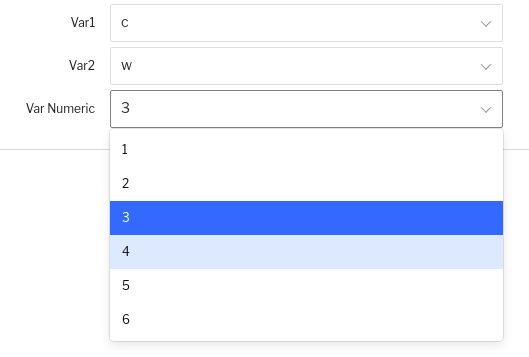To extend this functionality even further, you can perform multiple lookups to multiple different tables to create sequences of drop down variables where the options values change depending on upstream logic. This concept of implementing a "custom dynamic form" can be very useful. Situations where this can come in handy is say you have different protocols given the material of the part. You can construct tables that differentiate inputs based on material, and you could then query that table filtered by material.

Using drop down variables with tables provides an incredible amount of flexibility to customize how you implement "logic switches" in your pricing formulas. While the two examples I gave use `table_lookup` without any filtering conditions, you alternatively can use a `table_var` instance that lines out input values into a costing variable and filter by whatever column criteria you want.